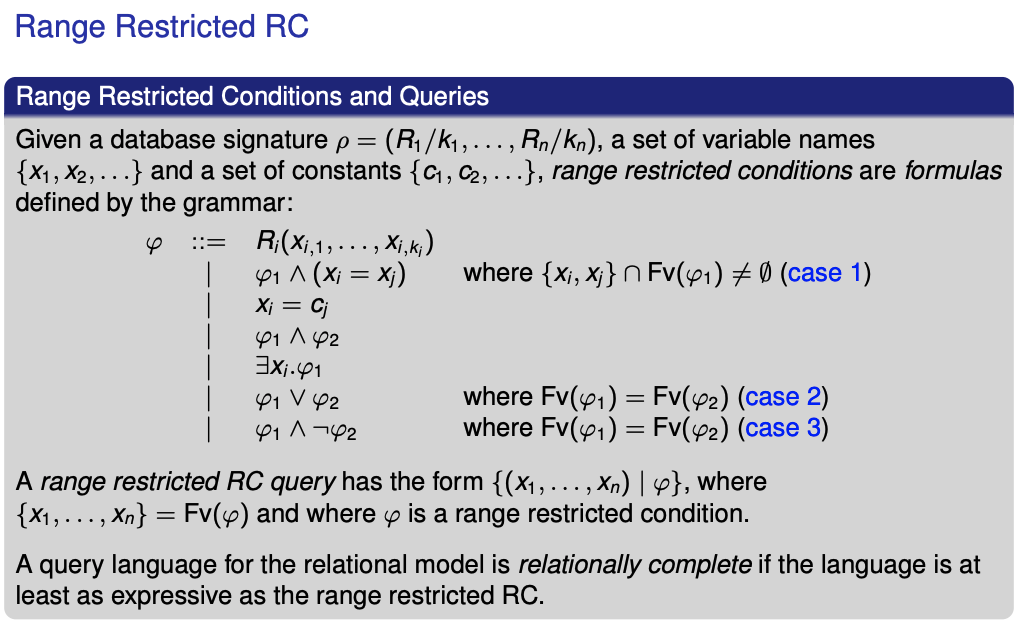Relational Database

# Relational Calculus (RC) §

Below are mainly confusing because of notation. In practice, just look at the examples, and it will be a lot clearer. However, it’s still good to know theory, see Theory vs. Practice.

#### Query Conditions §

The idea of query conditions is to use variables and valuations to generalize conditions.

• For example: AUTHOR(x, y) will be true of any valuation exactly when the 2-tuple of values occurs in AUTHOR.

Valuation

A valuation is a function that maps variable names to values in the universe: To denote a modification to in which variable is instead mapped to value , one writes:

These allow more complex conditions to be built from simpler conditions with logical connectives () and quantifiers ().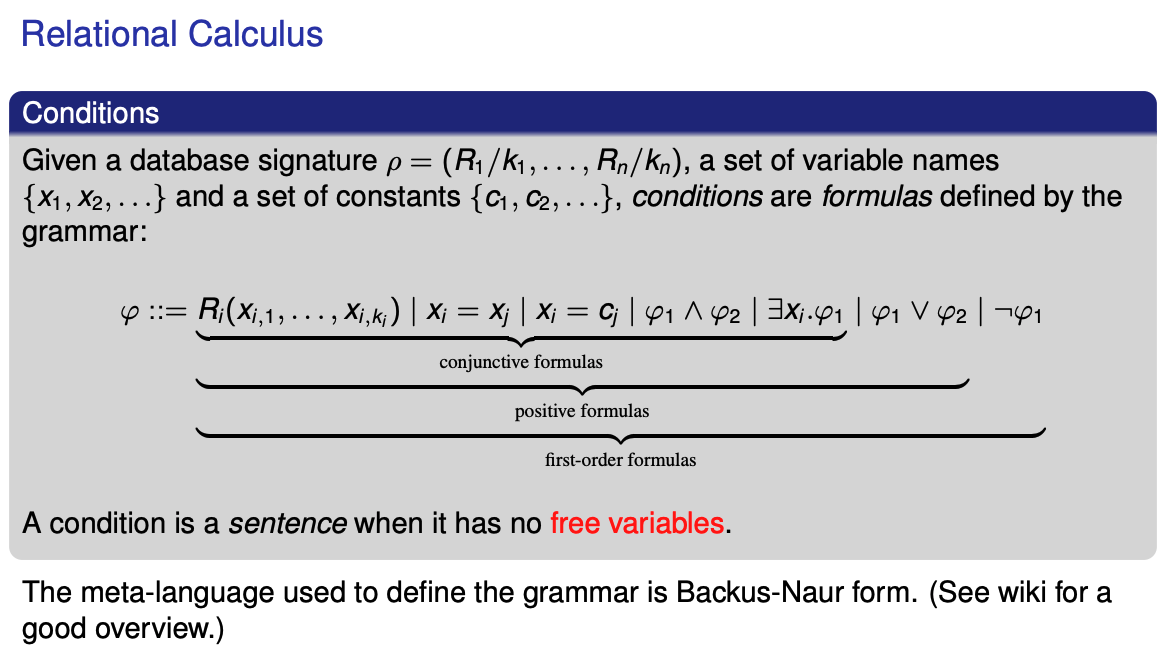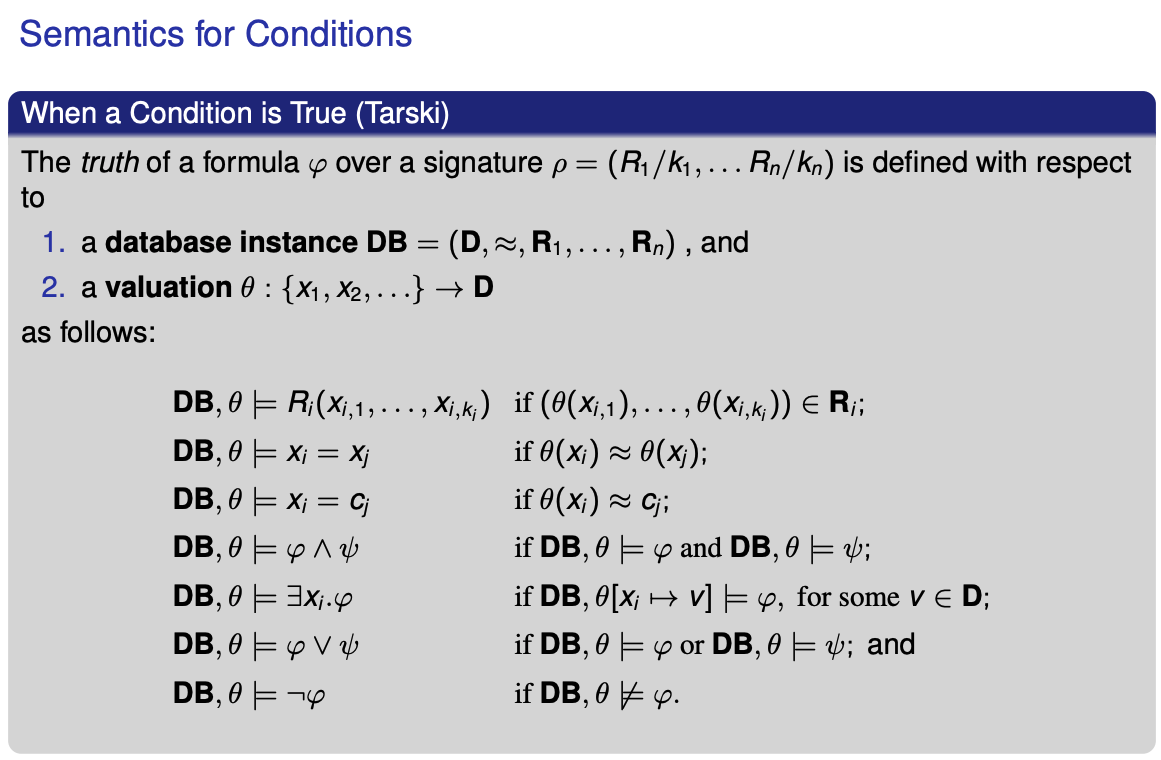#### Queries §

Relational Calculus (RC) Query

A query in the relational calculus is a set comprehension of the form where (are the free variables of ). Also:

• a conjunctive query is where is a conjunctive formula, and
• a positive query is where is a positive formula.

The answers to a query over is the relation

In other words, answers to queries are valuations applied to tuples of variables that make the formula true with respect to a database.

Some basic examples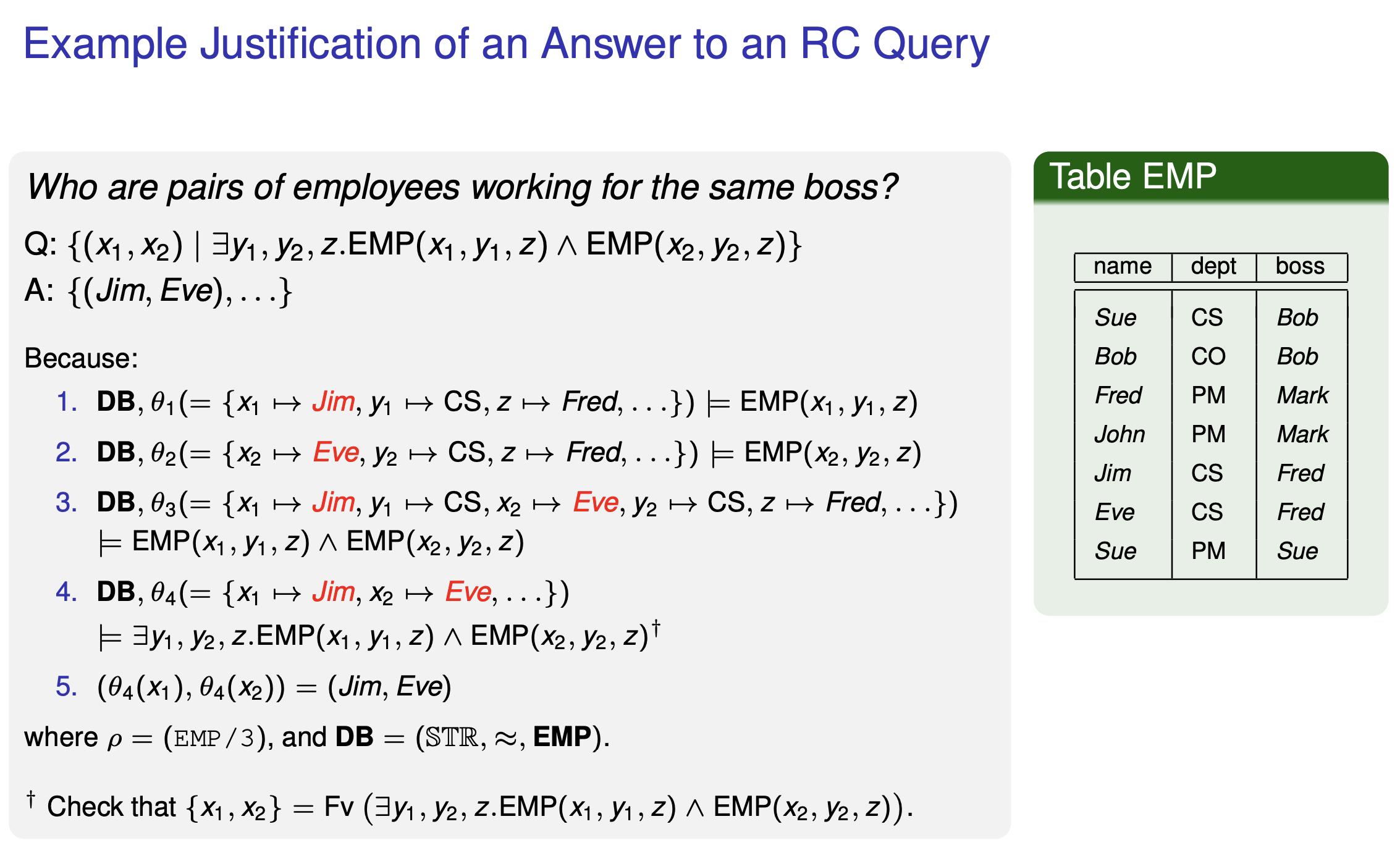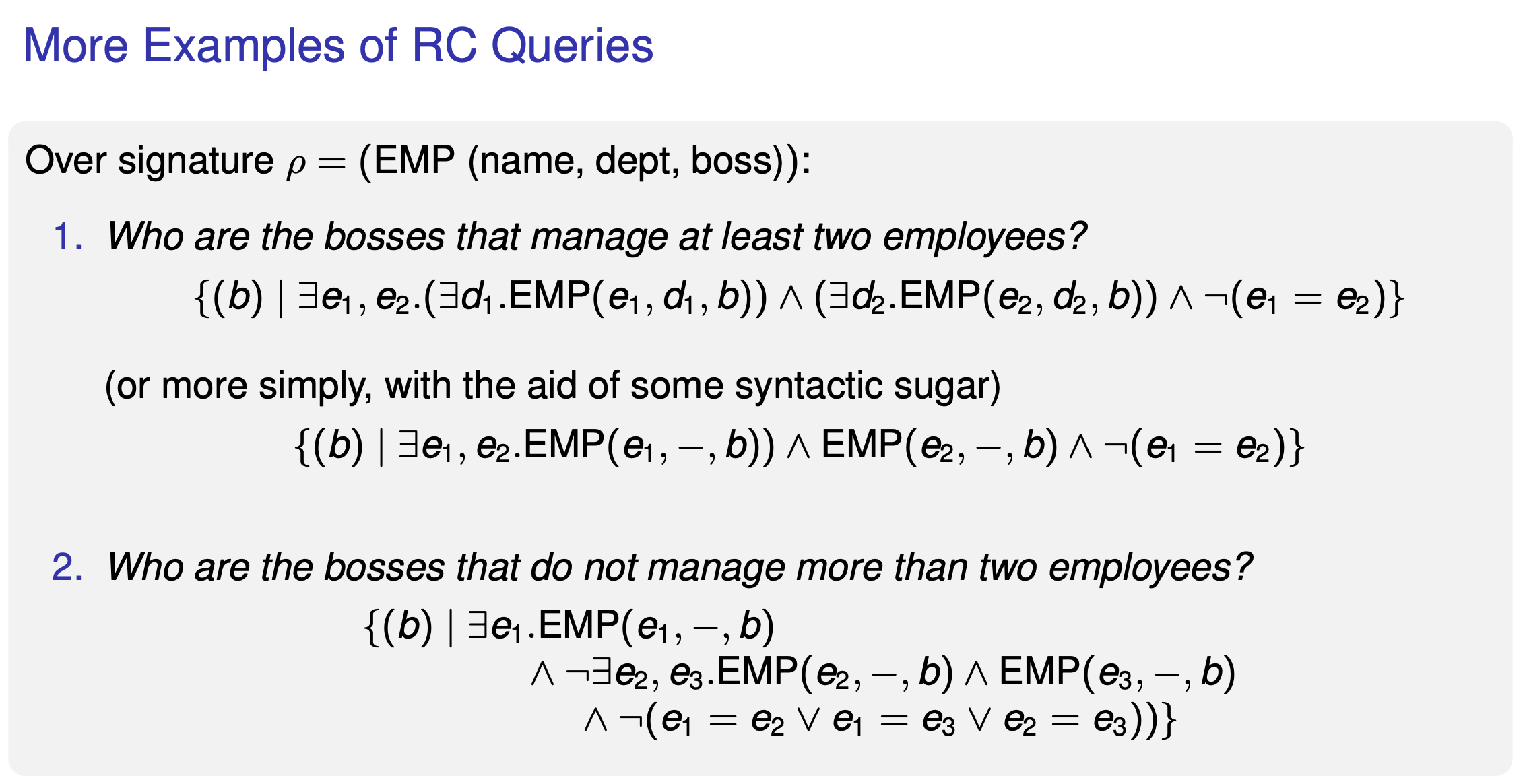### Safety §

Integrity Constraints aren’t enough for a RDBMS. We also need to have following properties:

• The extension of any relation in a signature should be finite
• Queries should be safe: their answers should be finite when database instances are finite

Look at the following queries, which would have infinite answers: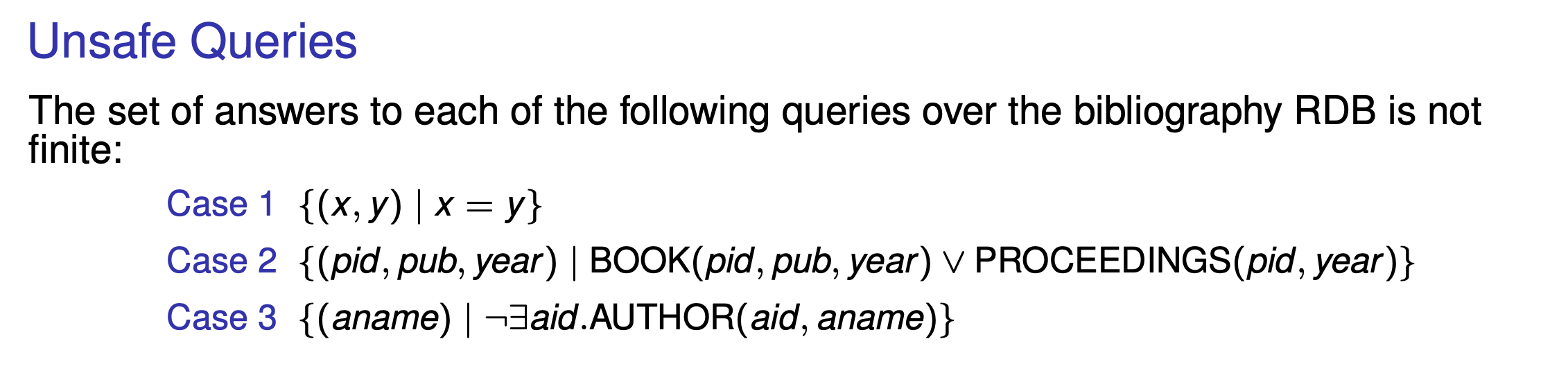Domain Independence

An RC query is domain independent when, for any pair of instances and and any if and only if

Theorem

Let be the signature of a relational database. Answers to domain independent queries contain only values that occur in the extension Ri of any relation .

safety ⇔ domain independence and finite database instances

Range-Restricted RC